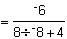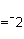# Order of Operations with Integers

In the last lesson we learned the rules for Order of Operations. We use the sentence: Please Excuse My Dear Aunt Sally to help us remember these rules:

 1 Simplify all operations inside Parentheses. 2 Simplify all Exponents, working from left to right. 3 Perform all Multiplications and Divisions, working from left to right. 4 Perform all Additions and Subtractions, working from left to right.

Until now we have worked with whole numbers only. Let's look at a few examples involving integers.

Example 1: Evaluate this arithmetic expression:  (-3)2 + -÷ -2

Solution:

 (-3)2 + -8 ÷ -2 = +9 + -8 ÷ -2 Simplify all exponents (Rule 2) +9 + -8 ÷ -2 = +9 + +4 Division (Rule 3) +9 + +4 = +13 Addition (Rule 4)

In Example 1, the parentheses are used to indicate multiplication. They make it clear that negative 3 is being multiplied by itself, or squared. The parentheses distinguish this problem from -1 times 3 squared.

(-3)2 = (-3)(-3) = +Negative three squared equals positive 9

-32 = -1(3)(3) = -1(+9) = -Negative one times three squared equals negative 9

Thus, when working with integers, it is a good idea to use parentheses to indicate multiplication. Let's look at some more examples.

Example 2: Evaluate this arithmetic expression:  (-8)(-5) ÷ 23

Solution:

 (-8)(-5) ÷ 23 = (-8)(-5) ÷ 8 Simplify all exponents (Rule 2) (-8)(-5) ÷ 8 = +40 ÷ 8 Perform all multiplications and divisions, working from left to right (Rule 3) +40 ÷ 8 = +5

In the next example, we will use both brackets [ ] and parentheses ( ). We will evaluate the expression from the innermost set of parentheses to the outermost set of brackets. Note that we are using the symbol · for multiplication instead of an "x" in the solution.

Example 3: Evaluate this arithmetic expression: 16 - [3(6 - 3) - 12]

Solution:

 16 - [3(6 - 3) - 12] = 16 - [3 · 3 - 12] Simplify all operations inside parentheses (Rule 1) 16 - [3 · 3 - 12] = 16 - [9 - 12] 16 - [9 - 12] = 16 - -3 16 - -3 = 16 + +3 = +19 Subtract (Rule 4)

In the next example, the fraction bar is used to indicate division. We must first evaluate each expression above and below the fraction bar. Then we can divide the numerator by the denominator.

Example 4: Evaluate this arithmetic expression:Solution:Summary: The rules for the order of operations are:

1. Simplify all operations inside Parentheses (and Brackets).
2. Simplify all Exponents, working from left to right.
3. Perform all Multiplications and Divisions, working from left to right. When working with fractions, evaluate each expression above and below the fraction bar. Then divide the numerator by the denominator.
4. Perform all Additions and Subtractions, working from left to right.

Tips: When evaluating arithmetic expressions with integers, we suggest that you:

1. Leave plenty of space on your paper for each problem.
2. Apply the rules for order of operations, working slowly and carefully.
3. Use parentheses to indicate multiplication.
4. Do not skip any steps since this may lead to careless errors.

### Exercises

Directions: Complete each exercise by applying the rules for order of operations. Click once in an ANSWER BOX and type in your answer; then click ENTER. After you click ENTER, a message will appear in the RESULTS BOX to indicate whether your answer is correct or incorrect. To start over, click CLEAR.

 1 18 - (-6)2 ÷ 9 ANSWER BOX:   RESULTS BOX:
 2 [49 + 3(4-9)] ÷ -17 ANSWER BOX:   RESULTS BOX:
 3 53 + (-9)(+2) ÷ 6 ANSWER BOX:   RESULTS BOX:
 4ANSWER BOX:   RESULTS BOX:
 5 (-4)(-3) ÷ 6 - 2[5 - -8 + (6 ÷ 2)] ANSWER BOX:   RESULTS BOX: